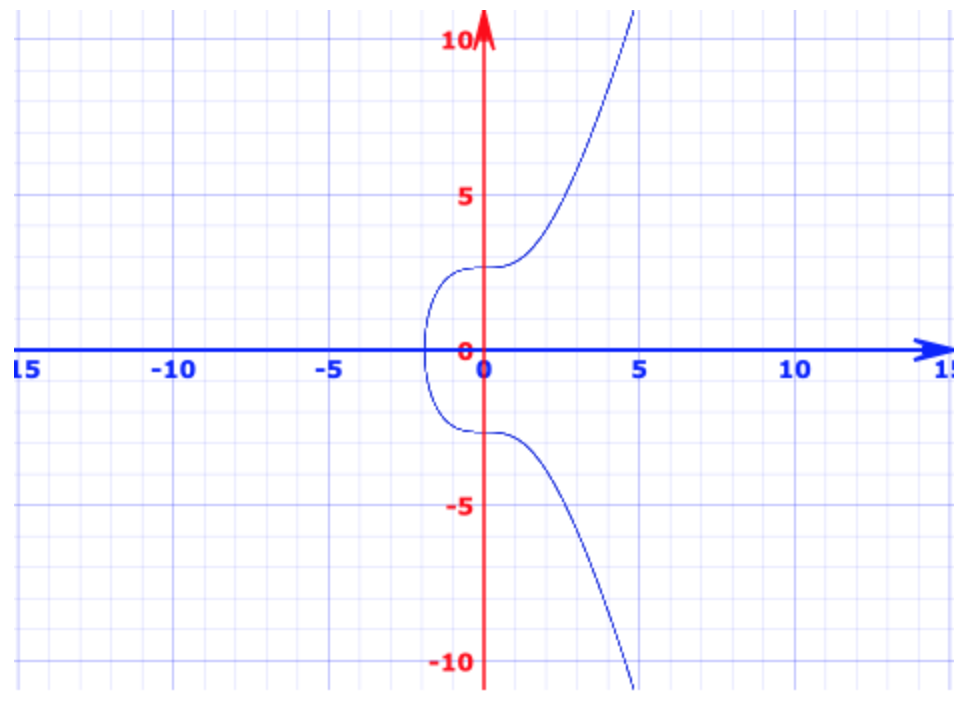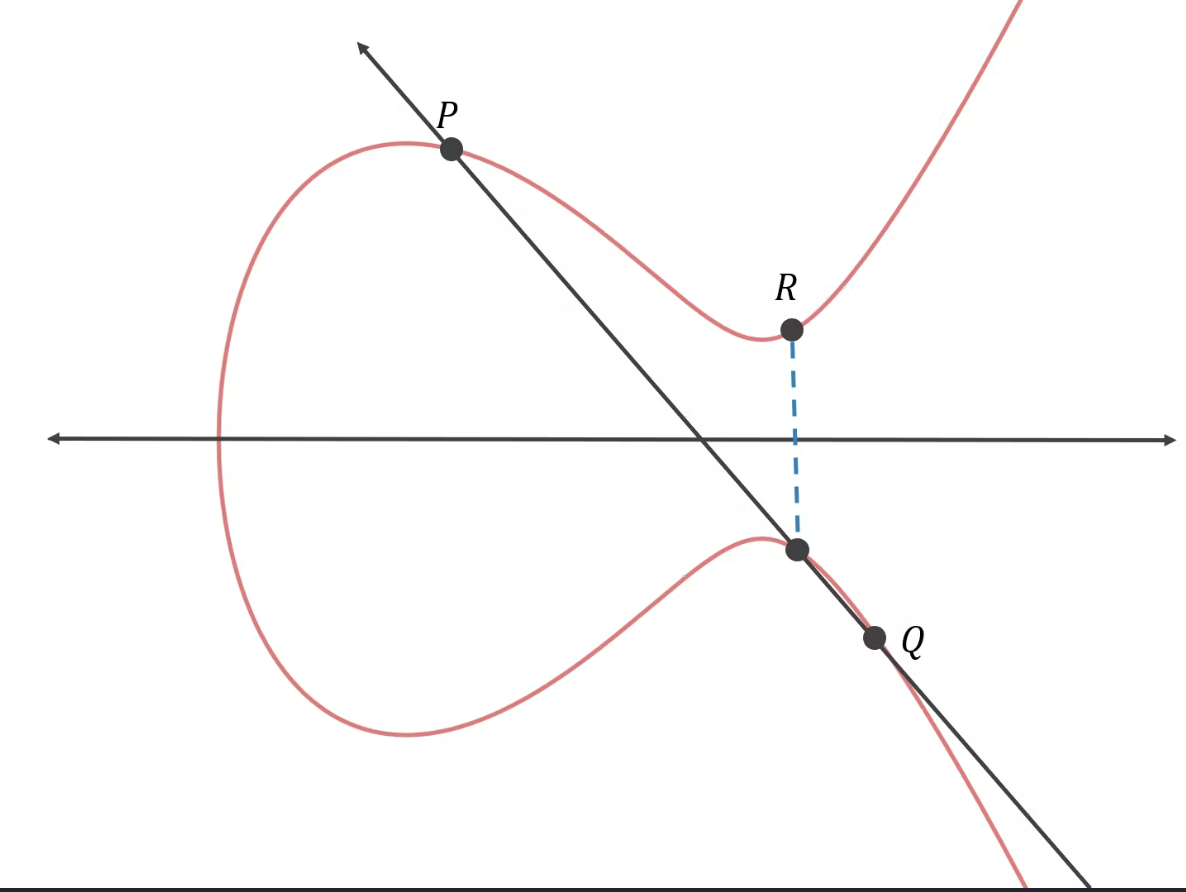# 非对称加密算法

## 前言

### 1、RSA非对称加解密的基本原理

a）基本假设与前提：两个大素数的乘积无法（轻易地）被因式分解
b）算法实现：
1）随机生成两个大的素数，p、q
2）令 n = p * q，另 N = （p-1) * (q-1)
3）从 在 (1, n-1）区间内，找到一个随机数d
4）找到一个 e，满足 e * d ≡ 1 mod N

ed ≡ 1 mod (p-1) * (q-1) , 由于 p-1 与 q – 1 分别与 n 互质 （最大公约数为1）， 所以 m ^ (ed) % n ≡ m ^ (1 % (q-1)*(p-1)) % n ≡ m % n (这句推导很魔幻，我看不懂，但大受震撼） , 从而解密过程无需知道p、q就能解密得到原文m。

### 2、DHKE 密钥交换的基本原理

DHKE严格上讲其实不是非对称加密算法，因为这个算法是通过非对称的方式来交换密钥，而最终通信双方使用相同的密钥来做对称的加解密。

A生成一个随机数a，B生成一个随机数b
A本地计算 d1 = g^a mod p
B本地计算 d2= g^b mod p
AB交换d1，d2（这时d1、d2可能被第三方窃取，但没关系）
A拿到d2计算 s = d2 ^ a mod p
B 拿到 d1 计算 s` = d1 ^ b mod p

s` = d1 ^ b mod p = (g ^ b ) ^ a mod p = g ^ ab mod p == s

### 3、Elliptic Curve 椭圆曲线非对称加密的基本原理

a) 首先，先弄明白什么是椭圆曲线。1）曲线是关于x轴对称的。
2）对曲线上任意两个非对称点做一条直线，该直线一定会和曲线相交与某一点
3）对曲线上任意一点（y = 0的点除外）做切线，该切线一定会和曲线相交与某一点

b）知道了曲线，我们定义一下曲线上的加法c）接下来定义私钥和公钥

d）加解密过程

Encrypt(m) = (a,b) = (r*G, m + r*X) ==> c
Decrypt(c) = b – x * a = m + r * x * G – x * r * G = m

e）补充说明